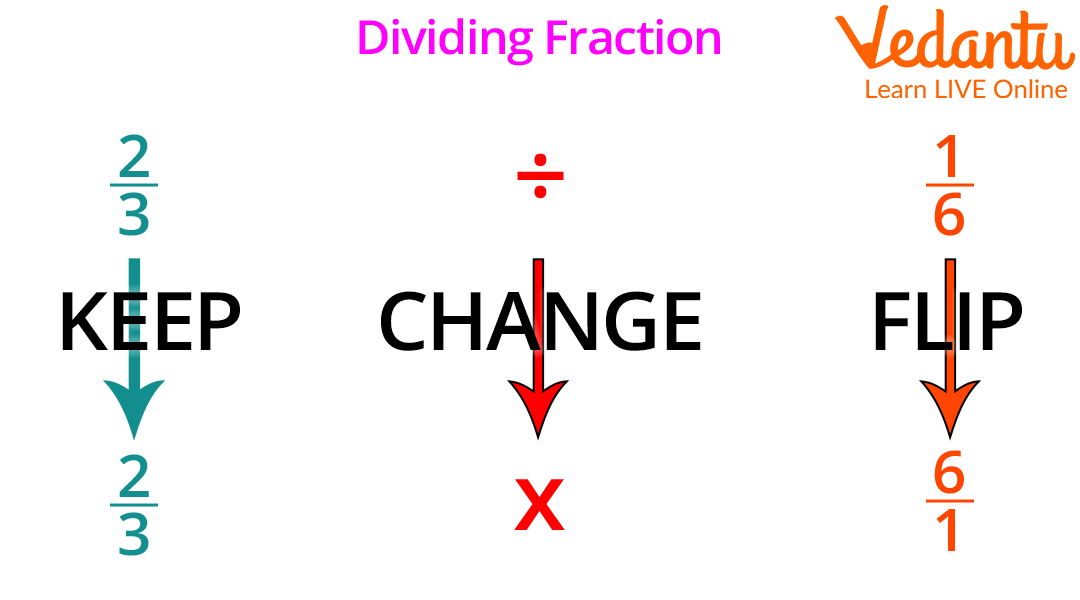Courses
Courses for Kids
Free study material
Free LIVE classes
More

# Division of FractionsLIVE
Join Vedantu’s FREE Mastercalss

## Introduction to Fractions

Before learning dividing fractions, let us first know what fractions are? Fractions are defined by division which represents the equal part of a whole. They are real numbers expressed in the form of $\frac{a}{b}$, where a and b are integers.

The numerator is the top number, written above the horizontal line, representing how many parts we have. The denominator is the bottom number, written below the horizontal line, representing how many equal parts the given item is splitted into. Hence, in the fraction $\frac{3}{4}$ (read as three fourths, and written as three-fourths), 3 is the numerator and 4 is the denominator. This tell us there are 3 parts, each of them is one-fourth (or $\frac{1}{4}$ ) of a whole.Fractions

Read below to know how to divide fractions.

### Division of Fractions with Unlike Denominators in 5 Easy Steps

As we have learnt what fractions are, now let us learn to divide fractions. Dividing fractions is similar to multiplying fractions. The only difference is that while dividing fractions, you need to flip the second fraction, i.e., divisor into a reciprocal and change the division sign to multiplication sign and multiply. Also, simplify if possible. Let us understand the steps with an example:Dividing Fractions

Example: What is $\frac{1}{2}\div\frac{1}{7}$ ?

Solution: The steps to divide the given fraction are as follows:

Step 1: Keep the first fraction and second fraction as it is given in the questions. This gives

$\frac{1}{2}\div\frac{1}{7}$

Step 2: Change the division symbol into a multiplication symbol.

$\frac{1}{2}\times \frac{1}{7}$

Step 3: Now, flip the second fraction or division into a reciprocal. To find the reciprocal, you just need to flip the numbers. That is, the numerator becomes the denominator and the denominator becomes the numerator. Accordingly, this gives:

$\frac{1}{2}\times \frac{7}{1}$

Step 4: Multiply the numerators and denominators of a fraction obtained in step 3. This gives:

$\frac{1}{2}\times \frac{7}{1} = \frac{7}{2}$

Step 5: Simply the result. Here, $\frac{7}{2}$ cannot be simplified further.

Therefore,  $\frac{1}{2}\times \frac{1}{7} = \frac{7}{2}$.

### Division of Fractions by a Whole Numbers

The steps to divide fractions by a whole number is the same as discussed above. The only difference is that the whole number here is converted into fractions by using the denominator as 1. Let us understand with an example:

Example: What is $\frac{5}{2}\div{4}$ ?

Solution: The steps to divide the given fraction are as follows:

Step 1: Keep the first fraction as it is given in the questions. Now, convert the whole number written after the division sign into a fraction by putting denominator equals to 1. Here the number is 4. This gives

$\frac{5}{2}\div\frac{4}{1}$

Step 2: Change the division symbol into a multiplication symbol.

$\frac{5}{2}\times \frac{4}{1}$

Step 3: Now, flip the second fraction or division into a reciprocal. Accordingly, this gives:

$\frac{5}{2}\times \frac{1}{4}$

Step 4: Multiply the numerators and denominators of a fraction obtained in step 3. This gives:

$\frac{5}{2}\times \frac{1}{4}=\frac{5}{8}$

Step 5: Simply the result. Here, 58 cannot be simplified further.

Therefore,  $\frac{5}{2}\div {4}=\frac{5}{8}$ .

### Division of Mixed Fractions

The steps to divide mixed fractions are the same as discussed above. The only difference is that the mixed number here is converted into improper fractions. Let us understand with an example:

Example: What is ${25}\frac{5}{2}\div {4}\frac{1}{4}$.

Solution:

Step 1: Change the mixed fractions into an improper fractions as shown below:

${25}\frac{5}{2}=\frac{25\times 2+5}{2} =\frac{55}{2}$

${4}\frac{1}{4}=\frac{4\times 4+1}{4} =\frac{17}{4}$

Now, ${25}\frac{5}{2}\div{4}\frac{1}{4} =\frac{55}{2}\div\frac{17}{4}$

Step 2: Keep the first fraction and second fraction as it is given in the questions. This gives:

$\frac{55}{2}\div\frac{17}{4}$

Step 3: Change the division symbol into a multiplication symbol.

$\frac{55}{2}\times\frac{17}{4}$

Step 4: Now, flip the second fraction or division into a reciprocal. Accordingly, this gives:

$\frac{55}{2}\times\frac{4}{17}$

Step 5: Multiply the numerators and denominators of a fraction obtained in step 3. This gives:

$\frac{55}{2}\times\frac{4}{17}=\frac{220}{34}$

Step 6: Simply the result. Here, $\frac{220}{34}$ can be simplified further as shown below:

Accordingly,  $\frac{{220}\div {2}}{{34}\div{2}}= \frac{110}{17}$

Therefore, ${25}\frac{5}{2}\div{4}\frac{1}{4} =\frac{110}{17} \:\text{or}\: {6}\frac {8}{17}$

### Dividing Fraction Song

Write the fractions to be divided

Flip the divisor and multiply

At the end, simplify the result.

So, the next time when you divide the fraction, don’t forget to follow the simple rule.

The simple rule is to flip and multiply.

In short, while dividing fractions, simply flip the second fraction or divisor into a reciprocal. Change the division sign into a multiplication sign. Multiply both numerator and denominator of a fraction. Simplify the result if possible.

Last updated date: 26th Sep 2023
Total views: 138.9k
Views today: 2.38k

## FAQs on Division of Fractions

1. How to divide fractions with the same denominator in an easy way?

While dividing fractions with the same denominator, you don’t need to flip the divisor and multiply. Rather, simply divide the numerator and denominator of the fraction individually to get the answer. Look at the example to understand it in a better way.  Here is the division of fractions with the same the denominator:

For example, $\frac{25}{7}\div\frac{5}{7} =\frac{{25}\div{5}}{{7}\div{7}}=\frac {5}{1}$.

2. What is the easiest way to remember the steps to divide fractions?

The easiest way to remember the steps to divide the fraction is to learn the phrase “Keep, Change, Flip, and Multiply”. The means keep the first fraction as it is, change the division sign into multiplication sign, flip the second fraction or divisor into a reciprocal, and multiply the fractions.